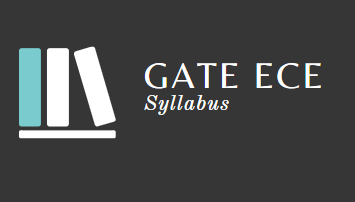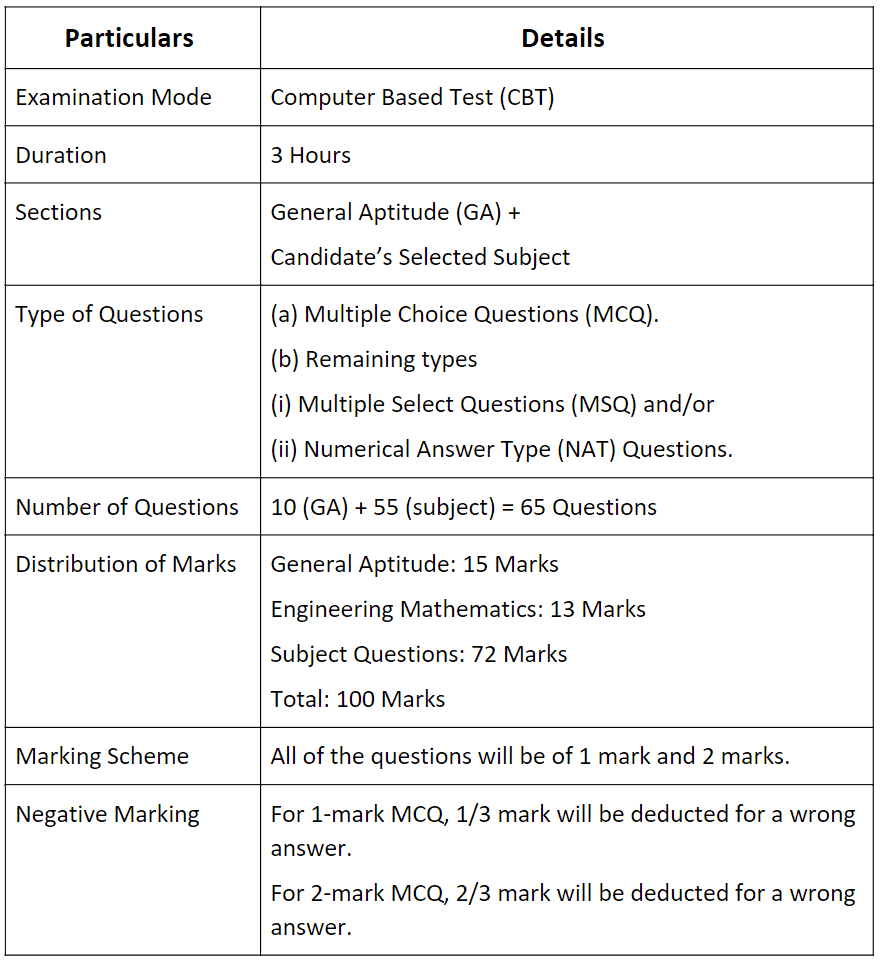GATE  >  Syllabus: Electronics and Communication Engineering (ECE), GATE 2023

# Syllabus: Electronics and Communication Engineering (ECE), GATE 2023 | GATE Past Year Papers for Practice (All Branches)

 Table of contentsIntroduction About GATE ECE 2023Topics & Sub-topics CoveredSyllabus for General Aptitude  (GA) (Common to all Papers)Electronics and Communication1 Crore+ students have signed up on EduRev. Have you?• Graduate Aptitude Test in Engineering (GATE) is a National-Level postgraduate engineering entrance exam for engineering graduate students. Usually, this is conducted by Indian Institute of Science, Bangalore and 7 other Indian Institute of Technology (IIT) located across India.
• It is conducted every year in the month of February and the next GATE entrance exam is expected to take place in the month of February 2023.
• This time it is IIT Kanpur that is going to conduct the GATE 2023. IIT Kanpur has released the syllabus for GATE Electronics and Communication Engineering 2023.
• Candidates who are appearing for GATE from Electronics and Communication Engineering discipline can refer the syllabus before they start their preparation.

Topics & Sub-topics Covered
Here is the list of topics and sub-topics for each subject that needs to be covered for the GATE 2023 Electronics & Communication Engineering Exam. Make sure the candidates get to know the following sections:

Candidates who are preparing for GATE 2023 Electronics and Communication Engineering Exam can check the syllabus given below before you start the preparation.

Before getting into the details of GATE 2023 Electronics and Communication Engineering Syllabus, let’s have an overview of GATE 2023 Exam Pattern for Electronics and Communication Engineering.

Table: Exam DetailsThe three sections of the GATE ECE paper are:
(i) General Aptitude
(ii) Engineering Mathematics
(iii) Subject-Specific Section

Syllabus for General Aptitude  (GA) (Common to all Papers)

1. Verbal Aptitude

• Basic English grammar: tenses, articles, adjectives, prepositions, conjunctions, verb-noun agreement, and other parts of speech.
• Basic vocabulary: words, idioms, and phrases in context.
• Narrative Sequencing

2. Quantitative Aptitude

• Data interpretation: data graphs (bar graphs, pie charts, and other graphs representing data), 2- and 3-dimensional plots, maps, and tables.
• Numerical computation and estimation: ratios, percentages, powers, exponents and logarithms, permutations and combinations, and series.
• Mensuration and Geometry
• Elementary Statistics and Probability

3. Analytical Aptitude

4. Spatial Aptitude

(a) Transformation of shapes:

(b) Paper folding
(c) Cutting

(d) Patterns in 2 and 3 dimensions.

Electronics and Communication

Section 1: Engineering Mathematics

• Linear Algebra: Matrix Algebra, Systems of linear equations, Eigenvalues, Eigenvectors.
• Calculus: Mean value theorems, Theorems of integral calculus, Evaluation of definite and improper integrals, Partial Derivatives, Maxima and minima, Multiple integrals, Fourier series, Vector identities, Directional derivatives, Line integral, Surface integral, Volume integral, Stokes’s theorem, Gauss’s theorem, Divergence theorem, Green’s theorem.
• Differential Equations: First order equations (linear and nonlinear), Higher order linear differential equations with constant coefficients, Method of variation of parameters, Cauchy’s equation, Euler’s equation, Initial and boundary value problems, Partial Differential Equations, Method of separation of variables.
• Complex Variables: Analytic functions, Cauchy’s integral theorem, Cauchy’s integral formula, Taylor series, Laurent series, Residue theorem, Solution integrals.
• Probability and Statistics: Sampling theorems, Conditional probability, Mean, Median, Mode, Standard Deviation, Random variables, Discrete and Continuous distributions, Poisson distribution, Normal distribution, Binomial distribution, Correlation analysis, Regression analysis.
• You can prepare Engineering Mathematics for GATE through EduRev’s Engineering Mathematics Course covering all the topics along with tests.

Section 2: & Signals and Systems

• Circuit analysis: Node and mesh analysis, superposition, Thevenin's theorem, Norton’s theorem, reciprocity. Sinusoidal steady state analysis: phasors, complex power, maximum power transfer. Time and frequency domain analysis of linear circuits: RL, RC and RLC circuits, solution of network equations using Laplace transform. Linear 2-port network parameters, wye-delta transformation.
• Continuous-time signals: Fourier series and Fourier transform, sampling theorem and applications.
• Discrete-time signals: DTFT, DFT, z-transform, discrete-time processing of continuous-time signals. LTI systems: definition and properties, causality, stability, impulse response, convolution, poles and zeroes, frequency response, group delay, phase delay.

Section 3: Electronic Devices

• Energy bands in intrinsic and extrinsic semiconductors, equilibrium carrier concentration, direct and indirect band-gap semiconductors.
• Carrier transport: diffusion current, drift current, mobility and resistivity, generation and recombination of carriers, Poisson and continuity equations.
• P-N junction, Zener diode, BJT, MOS capacitor, MOSFET, LED, photo diode and solar cell.

Section 4: Analog Circuits

• Diode circuits: clipping, clamping and rectifiers.
• BJT and MOSFET amplifiers: biasing, ac coupling, small signal analysis, frequency response. Current mirrors and differential amplifiers.
• Op-amp circuits: Amplifiers, summers, differentiators, integrators, active filters, Schmitt triggers and oscillators.

Section 5: Digital Circuits

• Number representations: binary, integer and floating-point- numbers.
• Combinatorial circuits: Boolean algebra, minimization of functions using Boolean identities and Karnaugh map, logic gates and their static CMOS implementations, arithmetic circuits, code converters, multiplexers, decoders.
• Sequential circuits: latches and flip-flops, counters, shift-registers, finite state machines, propagation delay, setup and hold time, critical path delay.
• Data converters: sample and hold circuits, ADCs and DACs.
• Semiconductor memories: ROM, SRAM, DRAM.
• Computer organization: Machine instructions and addressing modes, ALU, data-path and control unit, instruction pipelining.

Section 6: Control Systems

Section 7: Communications

• Random processes: autocorrelation and power spectral density, properties of white noise, filtering of random signals through LTI systems.
• Analog communications: amplitude modulation and demodulation, angle modulation and demodulation, spectra of AM and FM, superheterodyne receivers.
• Information theory: entropy, mutual information and channel capacity theorem.
• Digital communications: PCM, DPCM, digital modulation schemes (ASK, PSK, FSK, QAM), bandwidth, inter-symbol interference, MAP, ML detection, matched filter receiver, SNR and BER. Fundamentals of error correction, Hamming codes, CRC.

Section 8: Electromagnetics

• Maxwell's equations: ifferential and integral forms and their interpretation, boundary conditions,wave equation, Poynting vector.
• Plane waves and properties: reflection and refraction, polarization, phase and group velocity, propagation through various media, skin depth.
• Transmission lines: equations, characteristic impedance, impedance matching, impedance transformation, Sparameters, Smith chart.
• Rectangular and circular waveguides, light propagation in optical fibers, dipole and monopole antennas, linear antenna arrays.
The document Syllabus: Electronics and Communication Engineering (ECE), GATE 2023 | GATE Past Year Papers for Practice (All Branches) is a part of the GATE Course GATE Past Year Papers for Practice (All Branches).
All you need of GATE at this link: GATE

## GATE Past Year Papers for Practice (All Branches)

407 docs|127 tests

## GATE Past Year Papers for Practice (All Branches)

407 docs|127 tests

Track your progress, build streaks, highlight & save important lessons and more!

,

,

,

,

,

,

,

,

,

,

,

,

,

,

,

,

,

,

,

,

,

,

,

,

;